## Wednesday 9 November 2016

### Flare-on Challenge 2016 Write-up

The Flare-on challenge is an annual CTF style challenge with a focus on reverse engineering. Official solutions have already been published, besides that there are other writeups available too, hence I will just skim through the parts.

## Challenge #1

The first was simple. This is base64 encoding with a custom charset. This online tool does the job.
Flag: sh00ting_phish_in_a_barrel@flare-on.comFig 1: Challenge 1

## Monday 7 November 2016

### Hack the Vote 2016 CTF - APTeaser writeup

Just for fun I decided to have a go at the Hack the Vote 2016 CTF, particularly the reversing challenges on Windows. There were two of them APTeaser & Trumpervisor. I managed to solve the first. I did try the second but it involved reversing a Win 10 kernel driver implementing a hypervisor using the Intel Virtualization Extensions (VT-x). Anyway, here is a somewhat detailed writeup for the first.

## Initial Analysis

The provided file is a pcapng. Opening it in fiddler, reveals an interesting http request for a supposed pdf file on the domain important.documents.trustme, but as indicated from the Content-Type the response is actually an executable.Fig 1: Serving an executable when all I want is a pdf

## Sunday 30 October 2016

### A punched card reader in javascript

While trying out the Ektoparty CTF 2016 there was a challenge which requires to decode a series of punched card images. A punched card looks like this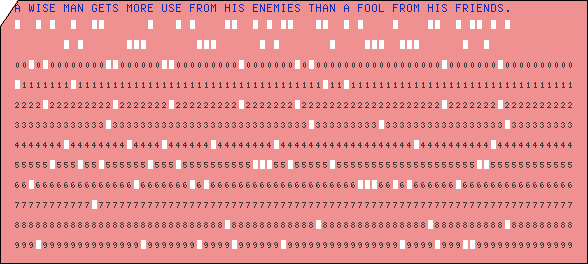Fig 1: A sample punch card

## Wednesday 7 September 2016

### Pyinstaller Extractor updated to v1.6

PyInstaller Extractor is a tool to extract the contents of a windows executable file created by pyinstaller. This weekend I updated the tool to version 1.6. The new features which were incorporated include

• Support for extracting pyinstaller 3.2
• Extractor would now use a random name for extracting unnamed files within the CArchive
• Preliminary support for handling encrypted pyz archives
The features are explained below.

## Support for extracting pyinstaller 3.2

There has not been any format changes between pyinstaller 3.2 and the earlier versions. The previous versions works as is for pyinstaller 3.2

## Handling unnamed files within CArchive

A Pyinstaller exe file can be visualized as of two nested archives. The outer layer is called CArchive. It is called so as it handled by C code i.e. the decompression of the layer is handled by a native stub written in C. The CArchive in turn contain another archive called PYZArchive along with other files. The PYZArchive is usually zlib compressed and is handled by python code and hence the reason for its name.

The CArchive usually contains the main script along with necessary dll files and python extension modules (pyd files). When running an pyinstaller exe, all dll and pyd files are written to a temporary directory to facilitate loading. (This behaviour is noticeably different from py2exe which loads dlls from memory.) The main script is never written to disk and hence it is possible to remove its name. If such is the case then the earlier versions of extractor would fail. The current version 1.6 will use a random name if it finds any unnamed files.

This feature has mainly been inspired while working on the PAN LabyREnth challenge (Threat #7).

## Preliminary support for handling encrypted pyz archives

The files within the PYZ archive can be encrypted too. If such is the case, the tool would dump those files as is without attempting to process them. Previously the tool would fail trying to decompress encrypted data.

That's it. I would make a separate post to demonstrate how to extract encrypted pyz archives. It isn't difficult as the key to decrypt is present right in the CArchive.

## Saturday 20 August 2016

### LabyREnth CTF WriteUp - Random track

Attempting the Labyrenth challenges was an interesting experience. I completed three tracks - Windows, Docs & Random, and the others were left halfway. Among all the tracks, the random track was more interesting particularly due to the last python challenge.

## Level 1 - Java

The challenge consists of a jar file. So it seems, we have to reverse java bytecode. The interesting thing is the jar will only run with Java 9 (currently in beta) as it uses StringConcatFactory. Attempting to decompile the file with Jad, Procyon, or CFR fails. This is due the fact it uses a new opcode InvokeDynamic. This is a fairly new opcode and as of now, the java compiler does not emit this opcode. It exists to support other dynamic languages running on the JVM.

Only krakatau was able to decompile the file proper, although it too doesn't handle the InvokeDynamic opcode.
``````public class omg {
String levelFlag;

public omg(String s)
{
super();
this.levelFlag = "W5SSA2DPOBSSA6LPOUQGK3TKN54SA5DINFZSASTBOZQSAYLQOAQGC4ZAO5QXE3JNOVYC4ICUNBSSA4TFON2CA53JNRWCAYTFEBWXKY3IEBWW64TFEBZWK6DDNF2GS3THEEQFI2DFEBTGYYLHEBUXGICQIFHHWRBQL5MTA5K7IV3DG3S7IJQXGZJTGJ6Q!";
if (s.contains((CharSequence)(Object)"-isDrunk"))
{
String[] a = s.split("-");
int i = a.charAt(2);
int i0 = Character.toUpperCase((char)i);
int i1 = (char)(i0 ^ 15);
this.levelFlag = /*invokedynamic*/null;
StringBuilder a0 = new StringBuilder(this.levelFlag);
int i2 = 0;
while(i2 < 4)
{
Object[] a1 = new Object;
int i3 = a.charAt(a.length() - 1);
int i4 = (short)i3;
a1 = Short.valueOf((short)i4);
int i5 = (char)(Integer.parseInt(String.format("%04x", a1), 16) ^ 166);
a0.append((char)i5);
i2 = i2 + 1;
}
System.out.println(a0.toString());
this.levelFlag = a0.toString();
}
else
{
int i6 = 0;
while(i6 < s.length() / 2)
{
String s0 = this.levelFlag;
int i7 = s.charAt(i6);
int i8 = s.charAt(s.length() - 1);
this.levelFlag = s0.replace((char)i7, (char)i8);
i6 = i6 + 1;
}
}
}

public String getLevelFlag()
{
return this.levelFlag;
}

public static void main(String[] a)
{
System.out.println(/*invokedynamic*/null);
}
}
``````
There is a whole bunch of decoy code. The actual flag  can be found by base32 decoding the levelFlag:

W5SSA2DPOBSSA6LPOUQGK3TKN54SA5DINFZSASTBOZQSAYLQOAQGC4ZAO5QXE3JNOVYC4ICUNBSSA4TFON2CA53JNRWCAYTFEBWXKY3IEBWW64TFEBZWK6DDNF2GS3THEEQFI2DFEBTGYYLHEBUXGICQIFHHWRBQL5MTA5K7IV3DG3S7IJQXGZJTGJ6Q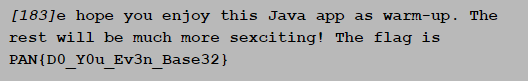Fig 1: Level 1 flag
This gives our flag: PAN{D0_Y0u_Ev3n_Base32}

## Level 2 - Regex

This challenge looks scary at first sight. A regular expression is given. Our task is to find a string that does not match the regex. Netcatting the string to 52.27.101.106 would give the flag.Fig 2: That looks scary, doesn't it?
The regex seems to match everything ever thrown at it. It has 625 clauses making it unfit for manual analysis. A regex debugger like RegexBuddy or regex101 is handy for such situations.

Lets look at the clause 1: .*[^0mglo8sc1enC3].*

This matches a single character unlimited number of times followed by a character which is not in the negation list, finally followed by a character unlimited number of times. Hence any string consisting of only characters present in the negation list will not match this clause.

Clause 2: .{,190}
Clause 3: .{192,}

The 2nd clause matches any string of length 190 or lower. The 3rd clause matches any string of length 192 or above. Combining these two clauses, for a non match our string should exactly 191 characters drawn from the list in clause 1.

The remaining clauses worth of interest is clause 124 and 341.Fig 3: Clause 124Fig 4: Clause 341
Clause 124 matches a string of length 190 chars followed by one of e0nlCo3c8. Similarly clause 341 matches a string of length 190 followed by one of mg1.

Combining the above clauses the string which does not match the regex consists of 190 g followed by a solitary s.
ggggggggggggggggggggggggggggggggggggggggggggggggggggggggggggggggggggggggggggggggggggggggggggggggggggggggggggggggggggggggggggggggggggggggggggggggggggggggggggggggggggggggggggggggggggggggggggggs

Netcatting this to 52.27.101.106 gives the flag PAN{th4t5_4_pr311y_dum8_w4y_10_us3_r3g3x}Fig 5: Level 2 flag

## Level 3 - Pcap

A pcap file is provided. Loading it in Wireshark reveals something interesting with the tcp sequence number of the SYN packets. The sequence numbers starts with PK\03\04 which is also the magic signature for zip files. We can set a display filter will only display the SYN packets. The same can be used to save the filtered packets to a new pcap file.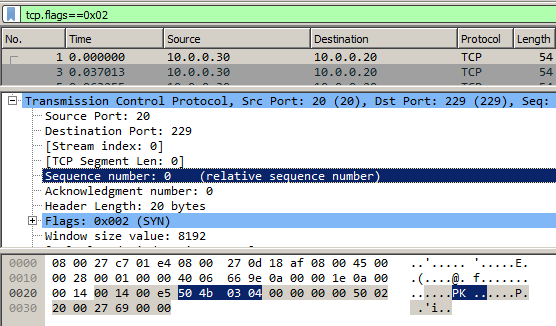Fig 6: ZIP signature in tcp sequence number
To assemble zip file by combining the sequence number, we can use scapy.
``````from scapy.all import *
from binascii import unhexlify
import cStringIO

def main():
buf = cStringIO.StringIO()
buf.write(unhexlify(format(pkt.seq, 'x').zfill(8)))
open('extracted.zip', 'wb').write(buf.getvalue())

if __name__ == '__main__':
main()
``````

This creates a new file extracted.zip. This zip contains 853 files each containing base64 encoded text.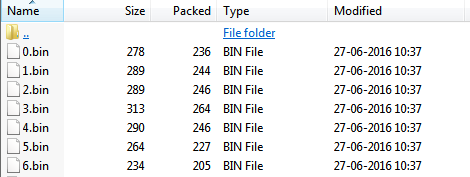Fig 7: Contents of zip file
Our task is to join the files in the correct order to form a huge base64 text blob. Decoding that text blob should give us our flag. The padding character used in base64 is =. Grepping for the = character within the extracted BIN files gives one hit 339.bin. This should therefore be the last file in the combined blob.Fig 8: 339.bin
The other point to note is that the contents of the files overlap as in Fig 9 showing the overlapped part of 531.bin with 339.bin.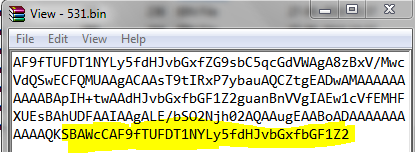Fig 9: Overlapped part
We can write a python script which would find the order of assembling the files by searching for the overlapped part.
``````import re

def findMatch(dct, txt):
for i in xrange(len(txt)-1, 0, -1):
reg = re.compile(re.escape(txt[0:i])+'\$')

for key in dct.keys():
if reg.search(dct[key]):
return (key, i)

return (None, -1)

def main():
contents = dict()

for i in xrange(853):
fname = '%d.txt' %i
contents[fname] = txt

nm = '339.txt'
print nm

while True:
txt = contents[nm]
result, matchlen = findMatch(contents, txt)

if result == None:
break

print result, matchlen
del contents[nm]
nm = result

if __name__ == '__main__':
main()
``````
Running this gives the order of joining the files in reverse i.e the first line (339.txt) is the last file and the line (659.txt) is the first file. Each line contains two comma separated values. The second value is the number of overlapping characters. The full list is available as a gist.

Based on the join order, another python script can assemble the files.
``````f = open('combined.txt', 'w')

try:
for line in reversed(lines):
fname, matchlen = line.split(' ')
matchlen = int (matchlen)

f.write(txt[0:len(txt)-matchlen])

except:
f.write(open('339.txt', 'r').read()) # last file has no overlap

f.close()
``````
The assembled file, combined.txt is a base64 text blob. Decoding it results in a zip file. The zip file has several images within.
One of them troll_cloud.png contains the flag:  PAN{YouDiD4iT.GREATjob}Fig 10: Level 3 flag

## Level 4 - PHP

The challenge consists of solving a maze. The maze is coded in PHP and is additionally heavily obfuscated. For running this, I had to set up wamp server in my windows virtual machine. The actual php code which we are after is dynamically generated and eval'd. Getting around this obfuscation was easy as PHP was configured with display errors to be true. This made it to spit out the eval'd php code as a warning message.Fig 11: Dynamically generated php code
To copy the de-obfuscated php code, we need to use a tool like fiddler. We cannot directly copy the php code from the warning message as the characters are not escaped properly. The deobfuscated source can be found here. From this point it is a matter of manual source code analysis to find the correct path for solving the maze. Solving the maze gives the flag:
PAN{Life is a maze of complications. Also, puppets are sometimes involved. Deal with it.}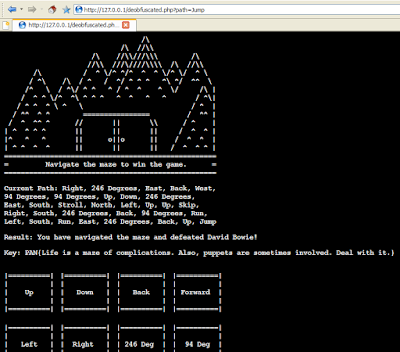Fig 12: Level  4 flag

## Level 5 - Python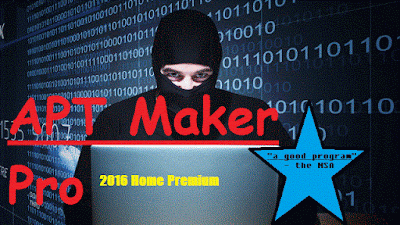Fig 13: Level 5 challenge - Crack APT Maker Pro
This the final challenge in the Random track and is the most interesting. As the description of the challenge points out we really "have to be a snake charmer to crack the newest version of APT Maker Pro". The file is a 720 KB python script which contains a zlib compressed marshalled code object which is dynamically executed by the exec statement as shown in Fig 14.Fig 14: Dynamically executed payload
To proceed, we need the decompiled payload from the compressed code object. This is easy and my tool Easy Python Decompiler is sufficient for the task. First, decompress the zlib data into a new file, add the python magic header 03 F3 0D 0A 00 00 00 00 at the beginning, and finally feed it to the decompiler to get our decompiled source as shown in Fig 15.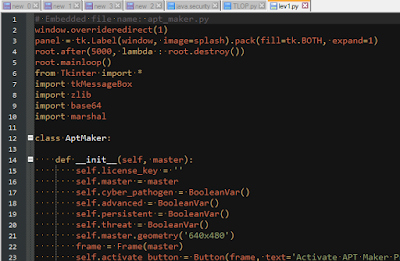Fig 15: Decompiled payload
However that is not all. The decompiled payload contains a base64 encoded, zlib compressed code object which is executed by the exec statement just like the previous case. In addition to this there is a piece of RC4 encrypted data labelled as malware as shown in Fig 16. Thus the actual challenge is to find the correct RC4 decryption key. To find the key, we need to inspect the second level payload just found.Fig 16: The malware blob
The difficulty at this stage increases rapidly. We cannot decompile the second level payload as it has been obfuscated. A deep knowledge about CPython internals is necessary to proceed forward. As I said, the payload is no longer decompilable, hence we need to inspect it manually. Let's inspect the code object.
``````>>> import marshal
>>> f = open('level2.pyc', 'rb')
>>> f.seek(8)
>>> co.co_consts
(<code object verify_license at 00AAB2F0, file "", line -1>, None)
``````
The code object contains another nested code object verify_license which sounds interesting. Lets dump it to a new file.
``````>>> of = open('level3.pyc', 'wb')
>>> of.write('\x03\xF3\x0D\x0A' + '\x00' * 4)
>>> marshal.dump(co.co_consts, of)
>>> of.close()
``````

Now we need to analyze 3rd level payload level3.pyc. Similar to level2.pyc this is too obfuscated and undecompileable. Lets run some preliminary analysis.
``````>>> f = open('level3.pyc', 'rb')
>>> f.seek(8)
>>> len(co.co_consts)
37173
>>> len(co.co_names)
37124
>>> len(co.co_code)
144060
``````
That's more than 37k constants and names!. In addition the size of bytecode instructions that gets actually executed is over 144k. Thats insane!. Who would like to analyze such a file manually unless one have tools and luckily we do have tools. Out of the 37173 constants, 37121 just store the None type and is redundant. We can write a quick python script for finding this.
``````>>> for i in xrange(len(co.co_consts)):
...     if co.co_consts[i] is not None:
...             print i
...             break
...
37121
``````
The 37122th constant stores a png file which looks interesting.
``````>>> co.co_consts[:6]
'\x89PNG\r\n'
``````Fig 17: Embedded PNG file
The remaining constants store various integers and are not of much interest. Lets shift our focus to the 144k long co_code. Lets disassemble the very first instruction,
``````>>> import opcode
>>> opcode.opname[ord(co.co_code)]
'EXTENDED_ARG'
``````

That's the EXTENDED_ARG opcode. In normal python, it is very rare to encounter this opcode. This is only generated if the operand of the instruction cannot fit in a space of 2 bytes. This can happen in rare situations such as passing more than 65,535 parameters to a function. The actual opcode on which EXTENDED_ARG is operating on is located at a offset of +3. Lets see what it is.
``````>>> opcode.opname[ord(co.co_code)]
'EXTENDED_ARG'
``````
That's even more strange. We expected to see a real opcode here. If we continue this way, we will see a huge chain of EXTENDED_ARG opcodes and the final instruction which it is operating on is a JUMP_FORWARD which as the name suggests increments the IP by an offset.
``````>>> opcode.opname[ord(co.co_code)]
'EXTENDED_ARG'
>>> opcode.opname[ord(co.co_code)]
'EXTENDED_ARG'
>>> opcode.opname[ord(co.co_code)]
'JUMP_FORWARD'
``````

To find out the target offset of the jump we need to write a python script.
``````import marshal

f=open('level3.pyc', 'rb')
f.seek(8)
f.close()

i = 0
arg = 0
while i < len(co.co_code):
arg = (arg << 16) | ord(co.co_code[i+1]) | (ord(co.co_code[i+2]) << 8)
arg = arg & 0xFFFFFFFF
i += 3

print hex(arg)
``````
Running this gives us the target offset which is 0xfffdcd45 or -1,44,059. That is instead of jumping forward it jumps backward within the instruction stream. The obfuscation that is applied is akin to overlapping instruction obfuscation found in native x86 executables.

Now the size of the instruction stream (co_code) is 144060 and a 144059 long backward jump from the rear leads to the second byte. If we disassemble this we uncover a hidden series of instructions stitched together with JUMP_FORWARDs.
``````>>> opcode.opname[ord(co.co_code)]
>>> opcode.opname[ord(co.co_code)]
'NOP'
>>> opcode.opname[ord(co.co_code)]
'JUMP_FORWARD'
``````

We need to uncover this hidden instructions, join them as one after removing the NOP and JUMP_FORWARD instructions used for stitching them. Another python script to the rescue.
``````# level3 extract code

import marshal
import opcode
import types

cleaned_bytecode = []

def clean(opkode, arg1, arg2):
if opcode.opname[opkode] == 'JUMP_FORWARD' or opcode.opname[opkode] == 'NOP':
return

else:
if opkode >= opcode.HAVE_ARGUMENT:
cleaned_bytecode.append(opkode)
cleaned_bytecode.append(arg1)
cleaned_bytecode.append(arg2)
else:
cleaned_bytecode.append(opkode)

def printline(offset, opname, arg):
if opname == 'JUMP_FORWARD' or opname == 'NOP':
return
if arg is not None:
print 'loc_%06d: %s %d' %(offset, opname, arg)
else:
print 'loc_%06d: %s' %(offset, opname)

def modifyCodeStr(code_obj):
co_argcount = code_obj.co_argcount
co_nlocals = code_obj.co_nlocals
co_stacksize = code_obj.co_stacksize
co_flags = code_obj.co_flags

# new code string
co_codestring = ''.join(map(chr, cleaned_bytecode))

# Replace png file contents to facilitate decompiling
co_constants = list(code_obj.co_consts)
co_constants = 'PNG FILE HERE'
co_constants = tuple(co_constants)

co_names = code_obj.co_names
co_varnames = code_obj.co_varnames
co_filename = code_obj.co_filename
co_name = code_obj.co_name
co_firstlineno = code_obj.co_firstlineno
co_lnotab = code_obj.co_lnotab

return types.CodeType(co_argcount, co_nlocals, co_stacksize, \
co_flags, co_codestring, co_constants, co_names, \
co_varnames, co_filename, co_name, co_firstlineno, co_lnotab)

def main():
f=open('level3.pyc', 'rb')
f.seek(8)
f.close()
kode = map(ord, list(co.co_code))
offset = 1

while offset < len(kode):
opkode = kode[offset]
opname = opcode.opname[opkode]

if opkode >= opcode.HAVE_ARGUMENT:
arg1 = kode[offset+1]
arg2 = kode[offset+2]
arg = (arg2 << 8) | arg1 # Little endian
printline(offset, opname, arg)
offset += 3

else:
arg = arg1 = arg2 = None
printline(offset, opname, arg)
offset += 1

clean(opkode, arg1, arg2)

if opname == 'JUMP_FORWARD':
offset += arg

elif opname == 'RETURN_VALUE':
break

newCodeObj = modifyCodeStr(co)
f = open('level3_deobf.pyc', 'wb')
f.write('\x03\xF3\x0D\x0A' + '\x00'*4)
marshal.dump(newCodeObj, f)
f.close()

if __name__ == '__main__':
main()
``````

The hidden instruction stream can be found here. The python script above stitches the hidden code and replaces the PNG file contents with a dummy string to facilitate decompiling, else decompiler would choke. Lets decompile the produced level3_deobf.pyc selecting pycdc as the engine. It gives the following code.
``````# File: l (Python 2.7)

= 'PNG FILE HERE'
exec       =    ==

= 'PNG FILE HERE'
exec       =    ==

= 'PNG FILE HERE'
exec       =    ==

= 'PNG FILE HERE'
exec       =    ==

= 'PNG FILE HERE'
exec       =    ==

= 'PNG FILE HERE'
exec       =    ==

= 'PNG FILE HERE'
exec       =    ==

= 'PNG FILE HERE'
exec       =    ==

= 'PNG FILE HERE'
exec       =    ==

= 'PNG FILE HERE'
exec       =    ==

= 'PNG FILE HERE'
exec       =    ==

= 'PNG FILE HERE'
exec       =    ==

= 'PNG FILE HERE'
exec       =    ==

= 'PNG FILE HERE'
exec       =    ==

= 'PNG FILE HERE'
exec       =    ==

= 'PNG FILE HERE'
exec       =    ==

= 'PNG FILE HERE'
exec       =    ==

= 'PNG FILE HERE'
exec       =    ==

= 'PNG FILE HERE'
exec       =    ==

= 'PNG FILE HERE'
exec       =    ==

= 'PNG FILE HERE'
exec       =    ==

= 'PNG FILE HERE'
exec       =    ==

= 'PNG FILE HERE'
exec       =    ==

= 'PNG FILE HERE'
exec       =    ==

= 'PNG FILE HERE'
exec       =    ==

=   &   &   &   &   &   &   &   &   &   &   &   &   &   &   &   &   &   &   &   &   &   &   &   &   &

``````
The variable names are missing, but it is fairly evident what the code does. It compares the characters of the license key with some bytes of the PNG file. For success, each of these checks must succeed. Joining the characters we get the  license key 1_W4nnA_b3_Th3_vERy_b3ST!. Feeding this, APT Maker Pro becomes registered as shown in Fig 18.Fig 18: APT Maker Pro is licensed
Clicking on Generate APT drops the malware payload as EVIL_MALWARE_ CYBER_PATHOGEN .pyc. Decompiling it we get the file containing the flag for this level
PAN{l1Ke_n0_oN3_ev3r_Wa5}Fig 19: The flag

## Tuesday 16 August 2016

### Solving the weasel keygenme by kao

Over at tuts4you kao posted a nice .net keygenme. The ultimate goal is developing a keygen which can generate keys for any name within a second.Fig 1: Know thy goal

Although the post is titled as "Solving the weasel keygenme", I did not get that far as developing a working keygen as required for the much coveted gold medal. However, the algorithm that kao implements is quite interesting to document and that is the reason for this blog post.

Solving the keygenme consists of two parts -- First to devirtualize the virtual machine and second to reverse the crypto.

## De-virtualizing the VM

Dropping the file in dnSpy, reveals that method and variable names have been removed as in Fig 2.Fig 2: Wazzup with those names?
The obfuscation can be removed by processing the file with de4dot which leads to a slightly better readable code as in Fig 3.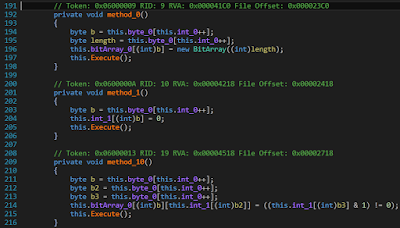Fig 3: Not anonymous anymore
Now with the obfuscation out of the way, it's time to deal with the vm. A vm based software protection works by transforming the original instructions to a set of new instruction which only a custom vm understands. Executing the new set of instructions under the supervision of the vm is expected to produce the same output as executing the original instructions without the vm. As regards to the implementation of a vm this keygenme is also no different.

The virtual machine is implemented in a class named SillyVM. The constructor of this class initializes the opcode handler table as shown in Fig 4. There are 16 instructions that the vm understands.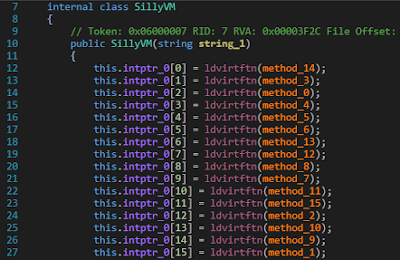Fig 4: Initializing the vm
Immediately, after setting up the opcode handler table the constructor initializes an array which contains the actual instructions of the vm as shown in Fig 5.Fig 5: The instructions of the vm
The next task is to understand the semantics of each of the 16 handlers. This is a manual task but it cannot be avoided. Understanding the operation of the handlers is crucial in comprehending the operation of the vm. Luckily, this is not a too difficult task, as the handlers are short and simple. The handlers can then be renamed as per their semantics. As shown in Fig 6, we have renamed three of the handlers to JumpIfLess, JumpIfZero, Multiply.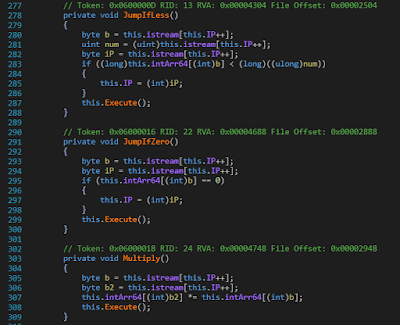Fig 6: Renaming the handlers
The complete set of handlers along with their associated semantics is listed in the following table.

INSTRUCTION OPCODE FORMAT SEMANTICS
JumpIfEqual 1 JumpIfEqual b1, b2, b3 If (arr[b1] == b2) then IP = b3
NewBitArray 2 NewBitArray b1, b2 bitarr[b1] = new BitArray(b2)
JumpIfLess 3 JumpIfLess b1, b2, b3 If (arr[b1] < b2) then IP = b3
JumpIfLargePassw 4 JumpIfLargePassw b1, b2 If (arr[b1] < passw.Length) then IP = b2
Jump 5 Jump b1 IP = b1
JumpIfZero 6 JumpIfZero b1, b2 If (arr[b1] == 0) then IP = b2
BitToInt 7 BitToInt b1, b2, b3 arr[b3] = (bitarr[b1][arr[b2]] == true ? 1 : 0)
Increment 8 Increment b1 arr[b1]++
IndexOf 9 IndexOf b1, b2 arr[b2] = hardc.IndexOf(passw[arr[b1]])
SumNumOne 10 SumNumOne b1, b2 arr[b2] = arr[b1] + 1
Multiply 11 Multiply b1, b2 arr[b2] *= arr[b1]
BitArrayCopy 12 BitArrayCopy b1 bitarr[b1].CopyTo(ans)
IntToBit 13 IntToBit b1, b2, b3 bitarr[b1][arr[b2]] = arr[b3] & 1
RShr 14 RShr b1 arr[b1] >>= 1
SetZero 15 SetZero b1 arr[b1] = 0

As per the above table, we can code a disassembler in python which takes in the instruction list and emits the de-virtualized C# code.
``````instr = [2,2,56,15,41,15,15,5,36,9,15,8,1,8,255,34,15,1,
5,30,13,2,41,8,8,41,14,8,8,1,3,1,5,20,8,15,4,15,
9,2,1,40,15,61,15,55,15,24,5,134,7,0,61,36,8,61,
15,18,5,78,7,0,61,19,6,19,74,7,2,18,19,0,19,36,8,
61,8,18,3,18,50,60,15,11,5,122,10,11,47,5,116,7,0,
61,19,6,19,112,7,2,11,19,7,2,47,57,11,19,57,0,57,
36,8,61,8,47,3,47,50,91,8,11,3,11,49,86,13,1,55,
36,8,55,8,24,3,24,40,50,12,1]

def disassemble():
ip = 0
while ip < len(instr):
opcode = instr[ip]
print 'loc_%03d:\t' %(ip),
ip += 1

if opcode == 0:
b1 = instr[ip]
b2 = instr[ip+1]
ip += 2
print 'arr[%d] += arr[%d];' %(b2, b1)

# JumpIfEqual
elif opcode == 1:
b1 = instr[ip]
b2 = instr[ip+1]
b3 = instr[ip+2]
ip += 3
print 'if (arr[%d] == (int)((sbyte)%d)) goto loc_%03d;' %(b1, b2, b3)

# NewBitArray
elif opcode == 2:
b1 = instr[ip]
b2 = instr[ip+1]
ip += 2
print 'bitarr[%d] = new BitArray(%d);' %(b1, b2)

# JumpIfLess
elif opcode == 3:
b1 = instr[ip]
b2 = instr[ip+1]
b3 = instr[ip+2]
ip += 3
print 'if ((long)arr[%d] < (long)((ulong)%d)) goto loc_%03d;' %(b1, b2, b3)

# JumpIfLargePassw
elif opcode == 4:
b1 = instr[ip]
b2 = instr[ip+1]
ip += 2
print 'if (arr[%d] < password.Length) goto loc_%03d;' %(b1, b2)

# Jump
elif opcode == 5:
b1 = instr[ip]
ip += 1
print 'goto loc_%03d;' %(b1)

# JumpIfZero
elif opcode == 6:
b1 = instr[ip]
b2 = instr[ip+1]
ip += 2
print 'if (arr[%d] == 0) goto loc_%03d;' %(b1, b2)

# BitToInt
elif opcode == 7:
b1 = instr[ip]
b2 = instr[ip+1]
b3 = instr[ip+2]
ip += 3
print 'arr[%d] = (bitarr[%d][arr[%d]] == true ? 1:0);' %(b3, b1, b2)

# Increment
elif opcode == 8:
b1 = instr[ip]
ip += 1
print 'arr[%d]++;' %(b1)

# IndexOf
elif opcode == 9:
b1 = instr[ip]
b2 = instr[ip+1]
ip += 2
print 'arr[%d] = "23456789ABCDEFGHJKLMNPQRSTUVWXYZ".IndexOf(password[arr[%d]]);' %(b2, b1)

# SumNumOne
elif opcode == 10:
b1 = instr[ip]
b2 = instr[ip+1]
ip += 2
print 'arr[%d] = arr[%d] + 1;' %(b2, b1)

# Multiply
elif opcode == 11:
b1 = instr[ip]
b2 = instr[ip+1]
ip += 2
print 'arr[%d] *= arr[%d];' %(b2, b1)

# BitArrayCopy
elif opcode == 12:
b1 = instr[ip]
ip += 1
print 'bitarr[%d].CopyTo(ans, 0);' %(b1)

# IntToBit
elif opcode == 13:
b1 = instr[ip]
b2 = instr[ip+1]
b3 = instr[ip+2]
ip += 3
print 'bitarr[%d][arr[%d]] = ((arr[%d] & 1) != 0);' %(b1, b2, b3)

# RShr
elif opcode == 14:
b1 = instr[ip]
ip += 1
print 'arr[%d] = (int)((uint)arr[%d] >> 1);' %(b1, b1)

# SetZero
elif opcode == 15:
b1 = instr[ip]
ip += 1
print 'arr[%d] = 0;' %(b1)

else:
print 'Error'
break

if __name__ == '__main__':
disassemble()
``````

Running the disassembler produces the following C# code.
``````loc_000: bitarr = new BitArray(56);
loc_003: arr = 0;
loc_005: arr = 0;
loc_007: goto loc_036;
loc_012: if (arr == -1) goto loc_034;
loc_016: arr = 0;
loc_018: goto loc_030;
loc_020: bitarr[arr] = ((arr & 1) != 0);
loc_024: arr++;
loc_026: arr = (int)((uint)arr >> 1);
loc_028: arr++;
loc_030: if ((long)arr < (long)((ulong)5)) goto loc_020;
loc_034: arr++;
loc_036: if (arr < password.Length) goto loc_009;
loc_039: bitarr = new BitArray(40);
loc_042: arr = 0;
loc_044: arr = 0;
loc_046: arr = 0;
loc_048: goto loc_134;
loc_050: arr = (bitarr[arr] == true ? 1:0);
loc_054: arr++;
loc_056: arr = 0;
loc_058: goto loc_078;
loc_060: arr = (bitarr[arr] == true ? 1:0);
loc_064: if (arr == 0) goto loc_074;
loc_067: arr = (bitarr[arr] == true ? 1:0);
loc_071: arr += arr;
loc_074: arr++;
loc_076: arr++;
loc_078: if ((long)arr < (long)((ulong)50)) goto loc_060;
loc_082: arr = 0;
loc_084: goto loc_122;
loc_086: arr = arr + 1;
loc_089: goto loc_116;
loc_091: arr = (bitarr[arr] == true ? 1:0);
loc_095: if (arr == 0) goto loc_112;
loc_098: arr = (bitarr[arr] == true ? 1:0);
loc_102: arr = (bitarr[arr] == true ? 1:0);
loc_106: arr *= arr;
loc_109: arr += arr;
loc_112: arr++;
loc_114: arr++;
loc_116: if ((long)arr < (long)((ulong)50)) goto loc_091;
loc_120: arr++;
loc_122: if ((long)arr < (long)((ulong)49)) goto loc_086;
loc_126: bitarr[arr] = ((arr & 1) != 0);
loc_130: arr++;
loc_132: arr++;
loc_134: if ((long)arr < (long)((ulong)40)) goto loc_050;
loc_138: bitarr.CopyTo(ans, 0);
``````

The de-virtualized code thus obtained is semantically equivalent to the original code, but is splattered with numerous gotos. This is because during virtualization the code lost its original structure. Loops were converted to If-then goto statements.  Although we can directly use the code as is, it is better if we try to restore it to its original form which should also improve readability.

## Restoring the structure of the de-virtualized code

To restore the original structure of the de-virtualized code we will compile it followed by decompilation with dnSpy.

Original de-virtualized code
``````void doIt()
{
bitarr = new BitArray;
bitarr = new BitArray(hardcoded);
arr = new int;
ans = new byte;

loc_000: bitarr = new BitArray(56);
loc_003: arr = 0;
loc_005: arr = 0;
loc_007: goto loc_036;
loc_012: if (arr == -1) goto loc_034;
loc_016: arr = 0;
loc_018: goto loc_030;
loc_020: bitarr[arr] = ((arr & 1) != 0);
loc_024: arr++;
loc_026: arr = (int)((uint)arr >> 1);
loc_028: arr++;
loc_030: if ((long)arr < (long)((ulong)5)) goto loc_020;
loc_034: arr++;
loc_036: if (arr < password.Length) goto loc_009;
loc_039: bitarr = new BitArray(40);
loc_042: arr = 0;
loc_044: arr = 0;
loc_046: arr = 0;
loc_048: goto loc_134;
loc_050: arr = (bitarr[arr] == true ? 1:0);
loc_054: arr++;
loc_056: arr = 0;
loc_058: goto loc_078;
loc_060: arr = (bitarr[arr] == true ? 1:0);
loc_064: if (arr == 0) goto loc_074;
loc_067: arr = (bitarr[arr] == true ? 1:0);
loc_071: arr += arr;
loc_074: arr++;
loc_076: arr++;
loc_078: if ((long)arr < (long)((ulong)50)) goto loc_060;
loc_082: arr = 0;
loc_084: goto loc_122;
loc_086: arr = arr + 1;
loc_089: goto loc_116;
loc_091: arr = (bitarr[arr] == true ? 1:0);
loc_095: if (arr == 0) goto loc_112;
loc_098: arr = (bitarr[arr] == true ? 1:0);
loc_102: arr = (bitarr[arr] == true ? 1:0);
loc_106: arr *= arr;
loc_109: arr += arr;
loc_112: arr++;
loc_114: arr++;
loc_116: if ((long)arr < (long)((ulong)50)) goto loc_091;
loc_120: arr++;
loc_122: if ((long)arr < (long)((ulong)49)) goto loc_086;
loc_126: bitarr[arr] = ((arr & 1) != 0);
loc_130: arr++;
loc_132: arr++;
loc_134: if ((long)arr < (long)((ulong)40)) goto loc_050;
loc_138: bitarr.CopyTo(ans, 0);
}
``````

Decompiled code after recompilation
``````// Token: 0x06000005 RID: 5 RVA: 0x00003A94 File Offset: 0x00002A94
private void doIt()
{
this.bitarr = new BitArray;
this.bitarr = new BitArray(this.hardcoded);
this.arr = new int;
this.ans = new byte;
this.bitarr = new BitArray(56);
this.arr = 0;
this.arr = 0;
{
if (this.arr != -1)
{
this.arr = 0;
while ((long)this.arr < 5L)
{
this.bitarr[this.arr] = ((this.arr & 1) != 0);
this.arr++;
this.arr = (int)((uint)this.arr >> 1);
this.arr++;
}
}
this.arr++;
}
this.bitarr = new BitArray(40);
this.arr = 0;
this.arr = 0;
this.arr = 0;
while ((long)this.arr < 40L)
{
this.arr = (this.bitarr[this.arr] ? 1 : 0);
this.arr++;
this.arr = 0;
while ((long)this.arr < 50L)
{
this.arr = (this.bitarr[this.arr] ? 1 : 0);
if (this.arr != 0)
{
this.arr = (this.bitarr[this.arr] ? 1 : 0);
this.arr += this.arr;
}
this.arr++;
this.arr++;
}
this.arr = 0;
while ((long)this.arr < 49L)
{
this.arr = this.arr + 1;
while ((long)this.arr < 50L)
{
this.arr = (this.bitarr[this.arr] ? 1 : 0);
if (this.arr != 0)
{
this.arr = (this.bitarr[this.arr] ? 1 : 0);
this.arr = (this.bitarr[this.arr] ? 1 : 0);
this.arr *= this.arr;
this.arr += this.arr;
}
this.arr++;
this.arr++;
}
this.arr++;
}
this.bitarr[this.arr] = ((this.arr & 1) != 0);
this.arr++;
this.arr++;
}
this.bitarr.CopyTo(this.ans, 0);
}
``````
[Full Code]
This can be further be hand optimized to convert all arrays to variables and while loops to for loop. The optimization will be done in a later step.

## The key checking algorithm

As shown in Fig 7 the keygenme expects a username/password combination. It calculates a standard MD5 hash of the username, and compares some bytes of it to another hash computed from the password. The algorithm which implements the latter hash is the crux of this keygenme. For success, these comparisons must match.Fig 7: Gimme the password!
The user supplied password is converted to a 50 size bit array using the following algorithm.
``````calc = new BitArray(50);

for (int i = 0, j = 0; i < password.Length; i++)
{
if (pos != -1)
{
for (int k = 0; k < 5; k++)
{
calc[j++] = (pos & 1) != 0;
pos >>= 1;
}
}
}
``````
Next, there is a huge hard coded byte array containing 6380 entries. This is also converted to a bit array. Since 8 bits make a byte, the size of the bit array is 6380 x 8 = 51,040.

Now, suppose the 50 bit password array is represented as p0, p1, p2, p3,......, p49. Using these two bit arrays, it calculates the value of 40 such following statements. The indices of the bits which are summed and multiplied are also fetched from the hard coded bit array. To understand how this is done refer to the decompiled code listing.
``````bit0 = (0 + p + p + p + ... + p + (p * p) + (p * p) + ... + (p * p)) & 1;
bit1 = (1 + p + p + p + ... + p + (p * p) + (p * p) + ... + (p * p)) & 1;
bit2 = (1 + p + p + p + ... + p + (p * p) + (p * p) + ... + (p * p)) & 1;
...
37 more lines
...
``````

The 40 calculated bits are then combined 8 at a time to form 5 bytes. For success, each byte thus obtained must match the corresponding byte obtained from the MD5 of the name as explained before.

## Converting to a boolean system

The algorithm the keygenme implements is a sort of combination of a system of linear & bilinear equations having 50 unknowns (p0, ...p49). Note that in each of the equations only the last bit of the sum is retained, the remaining bits are discarded. Hence this can be converted to a boolean system, but before that we need to convert the arithmetic addition and multiplication operations to boolean logic.

In a boolean system, variables can have two possible values viz 0 or 1. Further, since we are only interested in the last bit, the result of addition operation will either be 0 or 1. We can either use a  Karnaugh map or a truth table. Here I used the latter.

Truth Table for Converting Bit Addition
A B F = A + B
0 0 0
0 1 1
1 0 1
1 1 0

A & B are two bits. Bit B is added to Bit A. The rightmost column denotes the sum. Using the above truth table to convert it to a canonical sum-of-products boolean expression we get the following which is already in its simplest form,

Converting arithmetic product
Similar to addition, we can convert the product to an boolean expression,

Truth Table for converting Bit Product
A B C B.C F = A + B.C
0 0 0 0 0
0 0 1 0 0
0 1 0 0 0
0 1 1 1 1
1 0 0 0 1
1 0 1 0 1
1 1 0 0 1
1 1 1 1 0

Bit B & C are multiplied and added with Bit A. The rightmost column denotes the result. The canonical sum-of-products obtained is,

This can be further simplified to,

For simplification of boolean expressions,  there are online tools available such as http://www.32x8.com/ and http://tma.main.jp/logic/index_en.html.

## An attempt at keygenning

The objective of the keygenme is thus to solve a system of equations having 50 unknowns. Now, as I said before that the de-virtualized code can further be hand optimized to get rid of the excess clutter. Here is it.
``````private void doIt()
{
huge = new BitArray(hardcoded);
calc = new BitArray(50);
output = new BitArray(40);
arr = new int;
ans = new byte;

for (int i = 0, j = 0; i < password.Length; i++)
{
if (pos != -1)
{
for (int k = 0; k < 5; k++)
{
calc[j++] = (pos & 1) != 0;
pos >>= 1;
}
}
}

for (int x = 0, y = 0; x < 40; x++)
{
bool bit = huge[y++];

for (int a = 0; a < 50; a++)
if (huge[y++])
if (calc[a]) bit = !bit;

for (int b = 0; b < 49; b++)
for (int c = b + 1; c < 50; c++)
if (huge[y++])
if (calc[b] & calc[c]) bit = !bit;
output[x] = bit;
}
output.CopyTo(ans, 0);
}
``````
While loops have been converted to for loops. Arrays have been converted to variables. The last steps that remains is to develop a working keygen. For problems like this, I tend to prefer the Z3 SMT solver. Z3 has a nice python api to code against. Without further ado, here the is the code which tries to find a valid password for the name 0xec. It isn't a true keygen.
``````from z3 import *

from init_bits import initbits
from targ_bits import targbits
from sum_bits import sumbits
from prod_bits import prodbits

def main():
bits = [Bool('b'+str(i)) for i in range(50)]
s = SolverFor('sat') # Solver()
keyspace = '23456789ABCDEFGHJKLMNPQRSTUVWXYZ'
finalkey = ''
tempkey = ''

for i in range(len(initbits)):
A = BoolVal(initbits[i])

li = sumbits[i]
for x in li:
B = bits[x]
A = Or(And(Not(A), B), And(A, Not(B)))

li = prodbits[i]
for x, y in li:
B, C = bits[x], bits[y]
A = Or(And(A, Not(B)), And(A, Not(C)), And(Not(A), B, C))

print i

if s.check() == sat:
print 'Key Found!'
m = s.model()
for i in xrange(50):
val = m[bits[i]]
if val is not None:
tempkey += '1'
else:
tempkey += '0'

# Reverse tempkey
tempkey = tempkey[::-1]
for i in xrange(0, 50, 5):
finalkey +=  keyspace[int(tempkey[i:i+5], 2)]

finalkey = finalkey[::-1]
print finalkey[0:5] + '-' + finalkey[5:]

else:
print 'Could not find a key:('

if __name__ == '__main__':
main()
``````
Congrats if you have read this far, however, I have disappointing news in store. The keygen developed does not generate a key within a realistic time. For a gold medal, we need to generate a key within one second. I have ran the keygen for more than an hour, and it still continued running. However, I know the code is correct since it can verify the sample username / password combinations (kao/QQRR9-DL6JF).

Further optimizations of the python code are possible. but that doesn't improve the situation. For example, if you closely look at the boolean expression for converting bit addition, you can deduce that it is the same as a 2 bit half adder where we are discarding the carry bit.

Similarly, the expression for converting bit product is a 3 bit full adder discarding the carry.

However, in spite of all of the above optimizations, it still remains unsolved.

## Trying with an arithmetic system

As said before, the implemented algorithm is a combination of linear and bilinear system having 50 unknowns. In an arithmetic system, the problem looks like,

In simple maths, the above represents an equation similar to the following,

There are about 8 such equations. Each equation have a linear and a bilinear part. Without the bilinear part, we could have applied Gaussian Elimination for a solution, but that isn't the case to be. Further, my mathematical fu is not strong enough to devise an automated way to solve these type of equations. Hence, I will wait for others to solve this.

## Friday 10 June 2016

### Disk scheduling visualizer

Over the weekend, I developed a small python utility to visualize disk scheduling algorithms, particularly the way in which requests are serviced.

The algorithms implemented are SCAN & LOOK (not the circular versions i.e. CSCAN & CLOOK).

You can provide input, by modifying the below line in the code.
``````requestQ = [request(2, 0), request(156, 0), request(78, 0), request(192, 0),
request(19, 30), request(127, 30), request(90, 30), request(100, 150),
request(140, 150), request(60, 200)]```
```
Each of the entry is a named tuple consisting of the track number, and the time of request.

You can change the algorithm to look or scan by modifying the corresponding line `alg = AL_LOOK`

It will display a formatted table showing the way the requests are serviced.

Sample output

``````Track Range : 0 - 199
Starting Track : 89
Starting Direction : LOW
Algorithm : LOOK

TIME      |     TRACK
----------------|----------------
0        |       89
11       |       78
70       |       19
87       |       2
175       |       90
185       |      100
212       |      127
225       |      140
241       |      156
277       |      192
409       |       60```
```
Additionally, if you have matplotlib installed, it will render a graph too.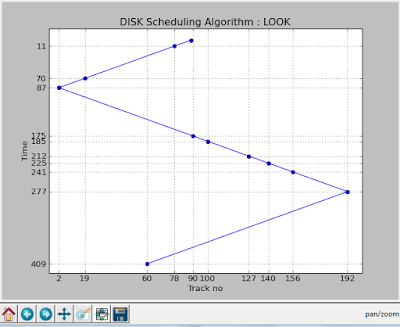Fig: Sample Screenshot

## Wednesday 11 May 2016

### Update (11-July-2017)

The project PjOrion Deobfuscator has been discontinued. This is superseded by bytecode_simplifier.

PjOrion is a python bytecode protector. While originally developed for obfuscating World of Tanks mods it can be used for pretty much any python code. What makes this protector special is that it works on the python bytecode itself. It tampers the bytecode making in un-decompilable and un-disassemble by standard tools.  For scripted languages like python, this is quite a significant improvement considering code protection in python was just a myth.

## An example

Some time in 2015 bomblader posted a crackme on tuts4you. I will be using the same crackme to demonstrate the protection offered by PjOrion.

PjOrion breaks existing disassemblers by tampering the bytecode. For example, using the standard dis module on the obfuscated pyc file results in the following output.

Not only did the disassembler fail but also there are several invalid opcodes in the listing. For example the opcode 144 is invalid and non existent. When cpython tries to execute an invalid opcode, it throws an exception. Without an exception handler installed the program would crash. This is precisely the reason why the very first instruction is SETUP_EXCEPT. The purpose of the instruction is to set up an exception handler at bytecode offset 102 which will be called when an exception is thrown.

It is clear that we need to follow the exception handler to understand the program flow. For this I developed a trivial program which could trace the program flow.

From the above listing, it is clear that in addition to the invalid instruction at the beginning the code is splattered with unconditional jumps. The result of this is a spaghetti control flow as shown in Fig 1 or an even more extreme example in Fig 2.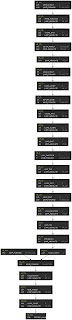Fig 1: Too many jumps!Fig 2: Devilish CFG

## Deobfuscating and beyond

To deobfuscate such files, I developed a tool PjOrion Deobfuscator (@github). It is currently in pre- alpha stage and may even not work. There are many moving pieces involved which needs refactoring to make this workable. With time I aim to improve this tool.

The tools removes redundant jumps between the basic blocks as in Fig 3. However this is not as simple as it sounds and needs to recursively disassemble the code stream. I also incorporated some ideas borrowed from the LLVM project to optimize the cfg.Fig 3: Redundant Jumps removal
After removing the redundant jumps, we need to reassemble the modified cfg. This is also quite a task as we need to re-compute all instruction offsets and the position of the basic blocks within the reassembled instruction stream.

For now, you can use the tool to generate a CFG which should help to better understand the bytecode. Be sure to have pydotplus and graphviz installed before using it.

I would like to reiterate once again that the tool is in a pre-alpha stage and may not work for your files. However, I definitely aim to improve this tool with time.

PjOrion Deobfuscator: https://github.com/extremecoders-re/PjOrion-Deobfuscator